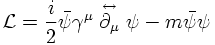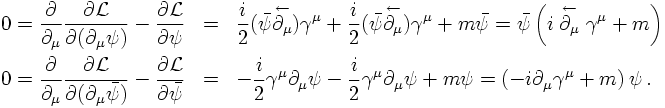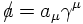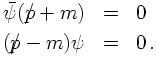## The Dirac equation

### The real thing

1. Charged spin-½ particles: Two spinors
2. Linearisation
3. Equation of motion for particle and antiparticle

EULER-LAGRANGE EQUATIONS FOR THE COMPLEX SCALAR FIELD

In order to obtain equations of motions (E.o.M.s) from free particle Lagrangians as the ones presented so far, functional derivatives w.r.t. the fields need to be evaluated. In the case of the complex scalar field, E.o.M.s for the field and its conjugate could be obtained by differentiating w.r.t. the conjugate field or the field itself, respectively: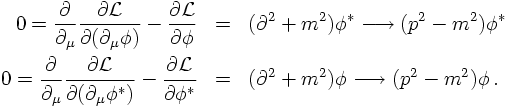EULER-LAGRANGE EQUATIONS FOR DIRAC-SPINORS

In the case of the spinor fields, the strategy will be similar. differentiating w.r.t. the undaggered/unbarred spinor will result in the E.o.M. for the daggerred/barred spinor and vice versa. For the Dirac-spinors with Lagrangian